# 6.4. Lesson: 空間統計¶

LinfinitiとS Motala（ケープ半島工科大学）が開発したレッスン

Spatial statistics allows you to analyze and understand what is going on in a given vector dataset. QGIS includes many useful tools for statistical analysis.

The goal for this lesson: To know how to use QGIS' spatial statistics tools within the Processing Toolbox.

## 6.4.1.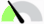Follow Along: テストデータセットの作成¶

We will create a random set of points, to get a dataset to work with.

To do so, you will need a polygon dataset to define the area you want to create the points in.

We will use the area covered by streets.

1. Start a new project

2. Add your `roads` dataset, as well as `srtm_41_19` (elevation data) found in `exercise_data/raster/SRTM/`.

注釈

You might find that the SRTM DEM layer has a different CRS to that of the roads layer. QGIS is reprojecting both layers in a single CRS. For the following exercises this difference does not matter, but feel free to reproject (as shown earlier in this module).

3. Open Processing toolbox

4. Use the Vector Geometry ▶ Minimum bounding geometry tool to generate an area enclosing all the roads by selecting `Convex Hull` as the Geometry Type: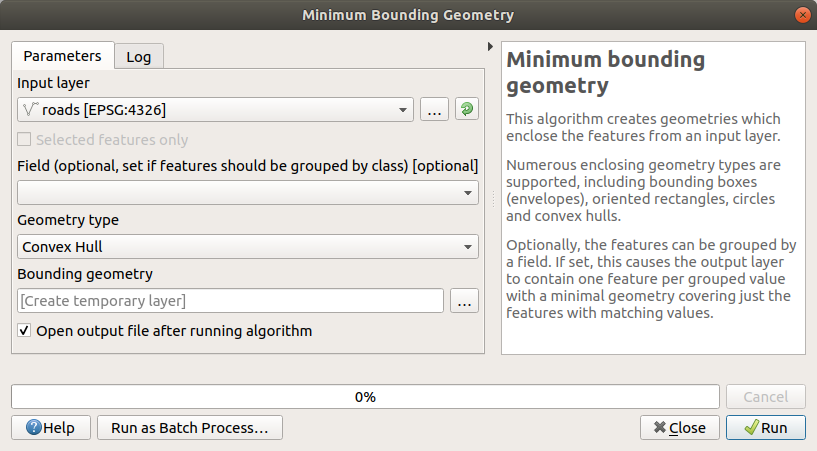As you know, if you don't specify the output, Processing creates temporary layers. It is up to you to save the layers immediately or at a later stage.

### 6.4.1.1. ランダム点群の作成¶

• Create 100 random points in this area using the tool at Vector Creation ▶ Random points in layer bounds, with a minimum distance of `0.0`: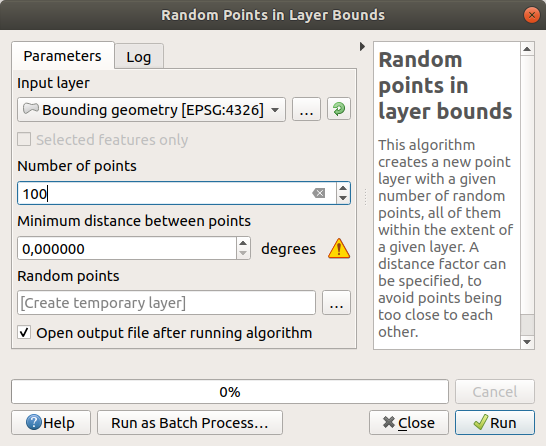注釈

The yellow warning sign tells you that that parameter concerns distances. The Bounding geometry layer is in a Geographical Coordinate System and the algorithm is just reminding you this. For this example we won't use this parameter so you can ignore it.

If needed, move the generated random point to the top of the legend to see them better: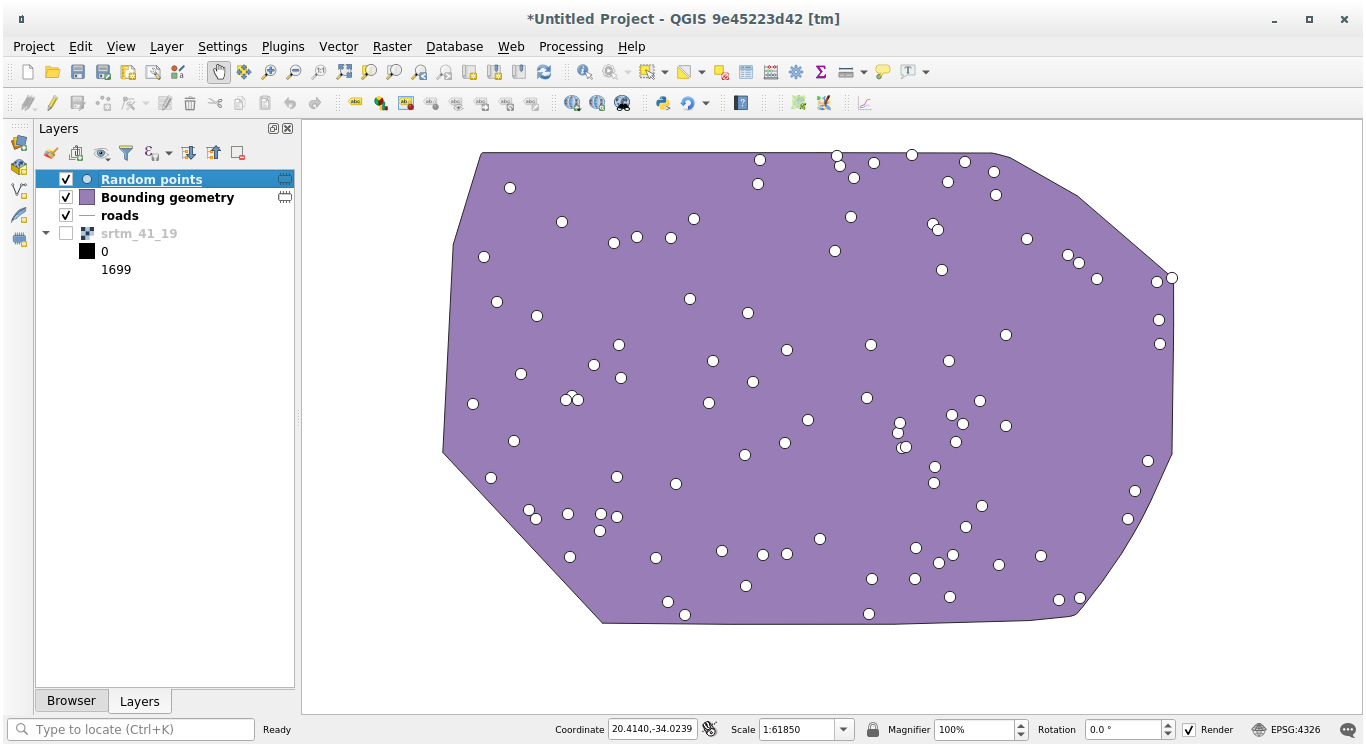### 6.4.1.2. データのサンプリング¶

To create a sample dataset from the raster, you'll need to use the Raster Analysis ▶ Sample raster values algorithm. This tool samples the raster at the locations of the points and adds the raster values in new field(s) depending on the number of bands in the raster.

1. Open the Sample raster values algorithm dialog

2. Select `Random_points` as the layer containing sampling points, and the SRTM raster as the band to get values from. The default name of the new field is `rvalue_N`, where `N` is the number of the raster band. You can change the name of the prefix if you want.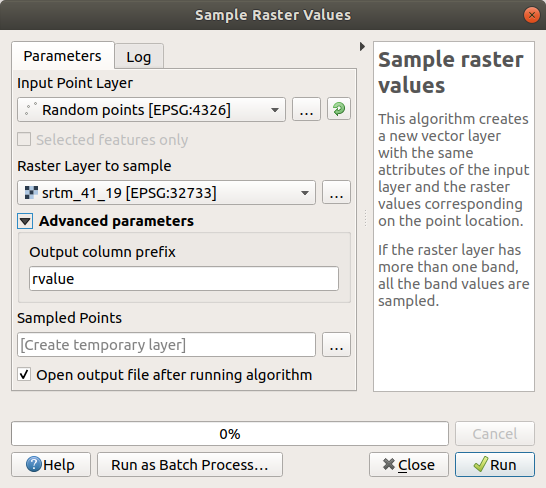3. Press Run

Now you can check the sampled data from the raster file in the attribute table of the `Sampled Points` layer. They will be in a new field with the name you have chosen.

サンプルレイヤーはここに示すとおりです: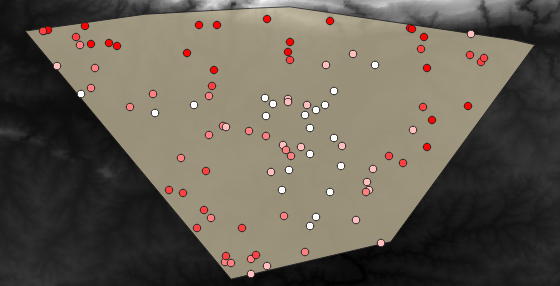The sample points are classified using the `rvalue_1` field such that red points are at a higher altitude.

You will be using this sample layer for the rest of the statistical exercises.

## 6.4.2.Follow Along: 基本統計¶

さて、このレイヤに対して基本統計を取得しましょう。

1. Click on the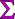Show statistical summary icon in the Attributes Toolbar. A new panel will pop up.

2. In the dialog that appears, specify the `Sampled Points` layer as the source.

3. Select the rvalue_1 field in the field combo box. This is the field you will calculate statistics for.

4. The Statistics Panel will be automatically updated with the calculated statistics: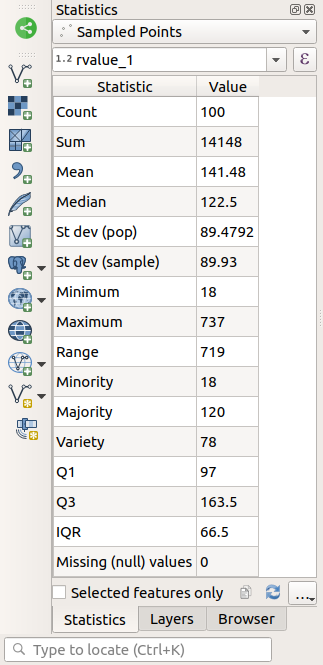注釈

You can copy the values by clicking on the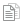Copy Statistics To Clipboard button and paste the results into a spreadsheet.

5. Close the Statistics Panel when done

Many different statistics are available:

カウント

The number of samples/values.

The mean (average) value is simply the sum of the values divided by the number of values.

If you arrange all the values from smallest to greatest, the middle value (or the average of the two middle values, if N is an even number) is the median of the values.

St Dev (pop)

Minimum

Maximum

レンジ

Q1

First quartile of the data.

Q3

Third quartile of the data.

Missing (null) values

The number of missing values.

## 6.4.3.Follow Along: Compute statistics on distances between points¶

1. Create a new temporary point layer.

2. Enter edit mode, and digitize three points somewhere among the other points.

Alternatively, use the same random point generation method as before, but specify only three points.

3. Save your new layer as distance_points in the format you prefer.

To generate statistics on the distances between points in the two layers:

1. Open the Vector Analysis ▶ Distance matrix tool.

2. Select the `distance_points` layer as the input layer, and the `Sampled Points` layer as the target layer.

3. このように設定します: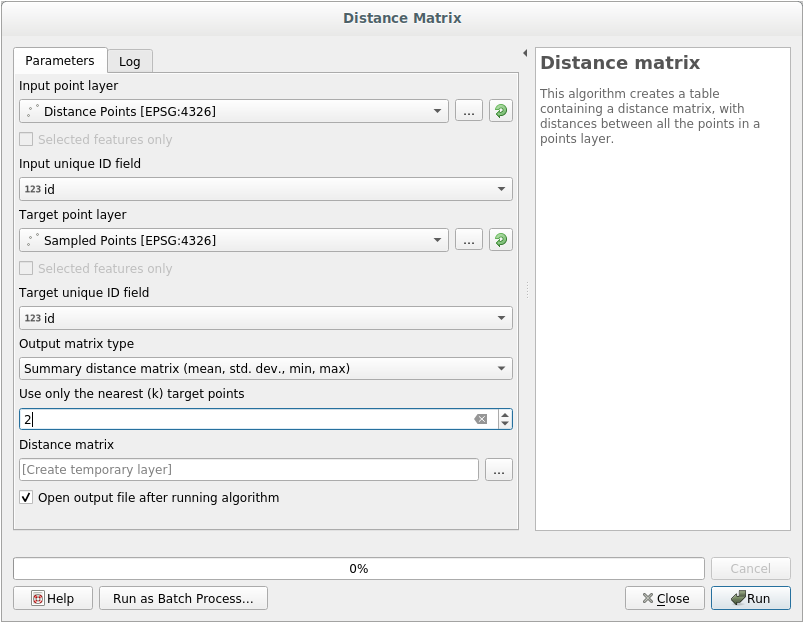4. If you want you can save the output layer as a file or just run the algorithm and save the temporary output layer later.

5. Click Run to generate the distance matrix layer.

6. Open the attribute table of the generated layer: values refer to the distances between the distance_points features and their two nearest points in the Sampled Points layer: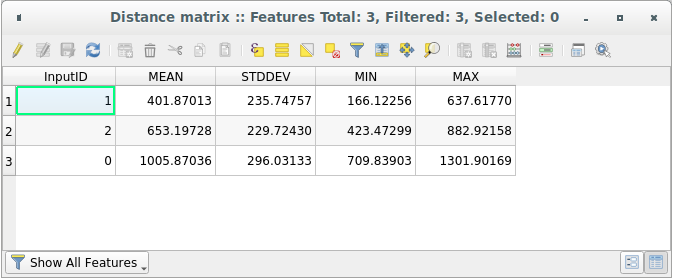With these parameters, the Distance Matrix tool calculates distance statistics for each point of the input layer with respect to the nearest points of the target layer. The fields of the output layer contain the mean, standard deviation, minimum and maximum for the distances to the nearest neighbors of the points in the input layer.

## 6.4.4.Follow Along: Nearest Neighbor Analysis (within layer)¶

To do a nearest neighbor analysis of a point layer:

1. Choose Vector analysis ▶ Nearest neighbor analysis.

2. In the dialog that appears, select the Random points layer and click Run.

3. The results will appear in the Processing Result Viewer Panel.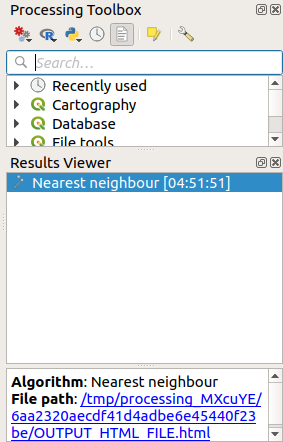4. Click on the blue link to open the `html` page with the results: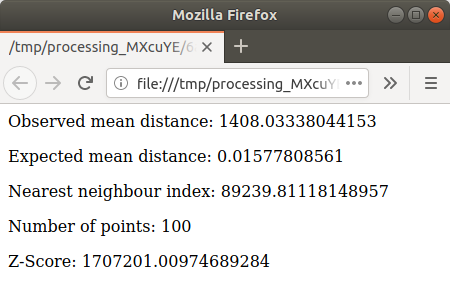## 6.4.5.Follow Along: 平均座標¶

データセットの平均座標を取得するために:

1. Start Vector analysis ▶ Mean coordinate(s)

2. In the dialog that appears, specify Random points as Input layer, and leave the optional choices unchanged.

3. 実行 をクリックします。

Let us compare this to the central coordinate of the polygon that was used to create the random sample.

1. Start Vector geometry ▶ Centroids

2. In the dialog that appears, select `Bounding geometry` as the input layer.

As you can see, the mean coordinates (pink point) and the center of the study area (in green) don't necessarily coincide.

The centroid is the barycenter of the layer (the barycenter of a square is the center of the square) while the mean coordinates represent the average of all node coordinates.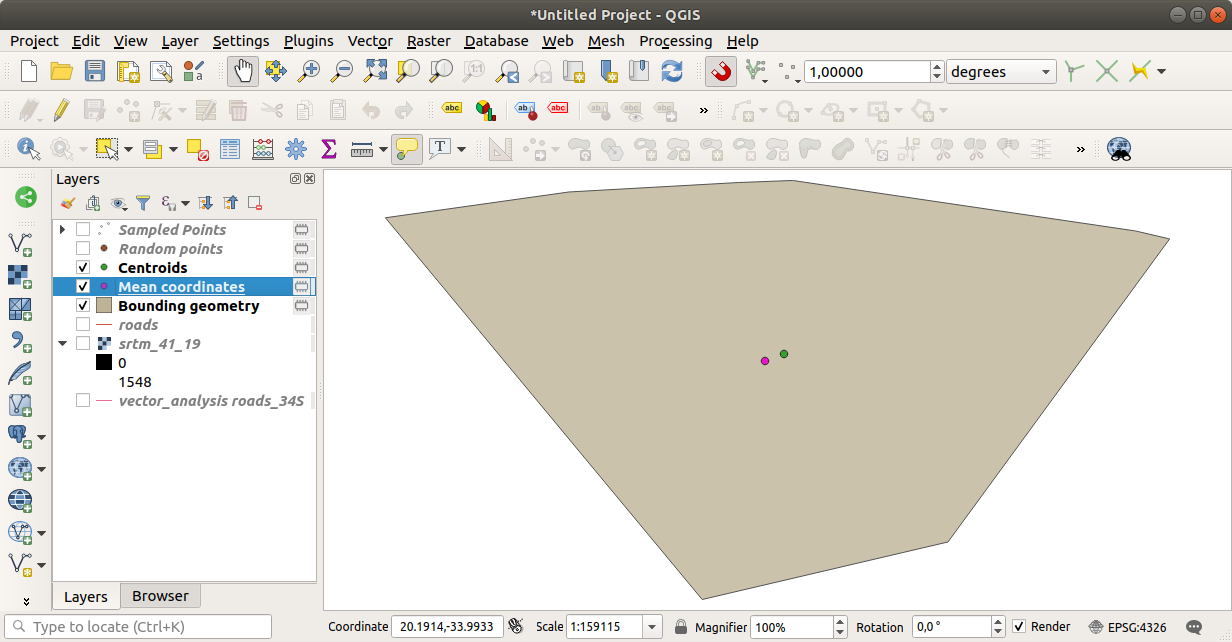## 6.4.6.Follow Along: 画像ヒストグラム¶

The histogram of a dataset shows the distribution of its values. The simplest way to demonstrate this in QGIS is via the image histogram, available in the Layer Properties dialog of any image layer (raster dataset).

1. In your Layers panel, right-click on the `srtm_41_19` layer

2. Select Properties

3. Choose the Histogram tab. You may need to click on the Compute Histogram button to generate the graphic. You will see a graph that shows the frequency distribution for the raster values.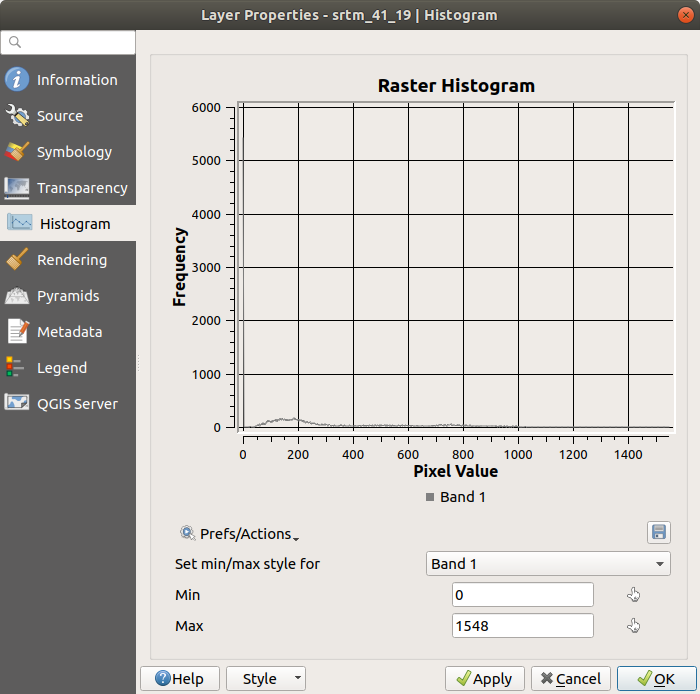4. The graph can be exported as an image with the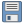Save plot button

5. You can see more detailed information about the layer in the Information tab (the mean and max values are estimated, and may not be exact).

The mean value is `332.8` (estimated to 324.3), and the maximum value is `1699` (estimated to 1548)! You can zoom in the histogram. Since there are a lot of pixels with value `0`, the histogram looks compressed vertically. By zooming in to cover everything but the peak at `0`, you will see more details: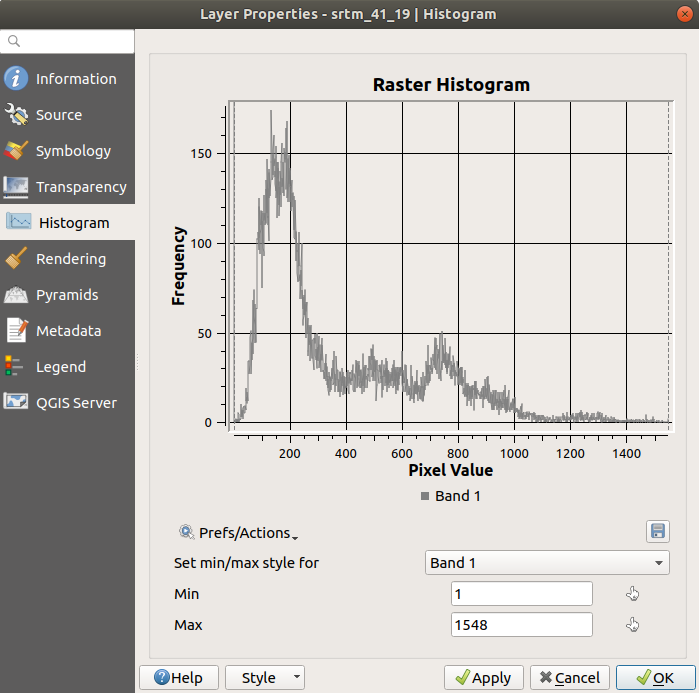If the mean and maximum values are not the same as above, it can be due to the min/max value calculation. Open the Symbology tab and expand the Min / Max Value Settings menu. Choose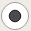Min / max and click on Apply.

Keep in mind that a histogram shows you the distribution of values, and not all values are necessarily visible on the graph.

## 6.4.7.Follow Along: 空間的補間¶

Let's say you have a collection of sample points from which you would like to extrapolate data. For example, you might have access to the Sampled points dataset we created earlier, and would like to have some idea of what the terrain looks like.

1. To start, launch the GDAL ▶ Raster analysis ▶ Grid (IDW with nearest neighbor searching) tool in the Processing Toolbox.

2. For Point layer select `Sampled points`

3. Set Weighting power to `5.0`

4. In Advanced parameters, set Z value from field to `rvalue_1`

5. Finally click on Run and wait until the processing ends

6. Close the dialog

Here is a comparison of the original dataset (left) to the one constructed from our sample points (right). Yours may look different due to the random nature of the location of the sample points.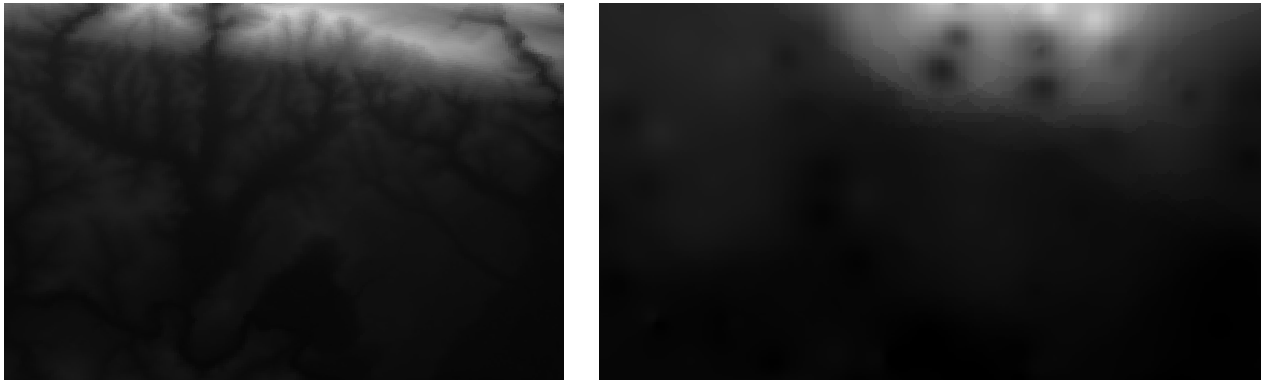As you can see, 100 sample points aren't really enough to get a detailed impression of the terrain. It gives a very general idea, but it can be misleading as well.

## 6.4.8.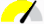Try Yourself Different interpolation methods¶

1. Use the processes shown above to create a set of 10 000 random points

注釈

If the number of points is really big, the processing time can take a long time.

2. Use these points to sample the original DEM

3. Use the Grid (IDW with nearest neighbor searching) tool on this dataset.

4. Set Power and Smoothing to `5.0` and `2.0`, respectively.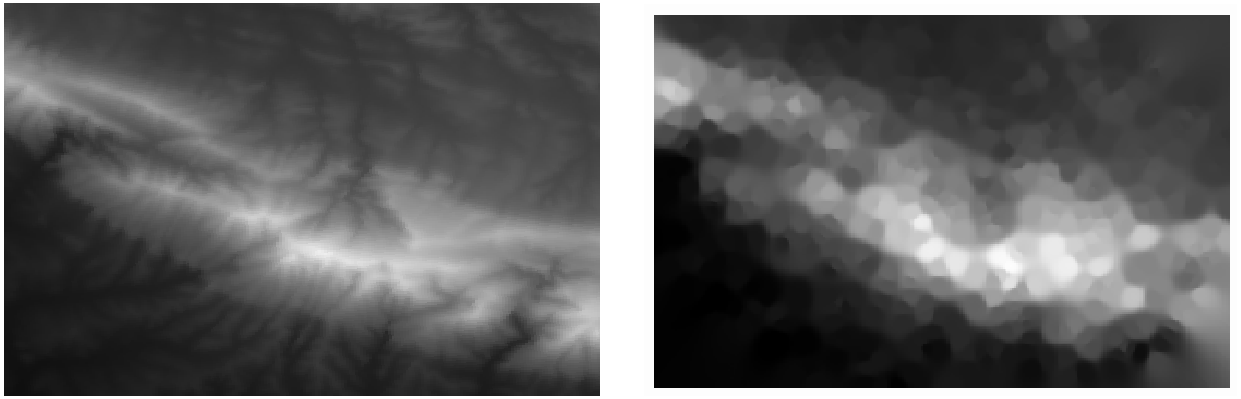This is a better representation of the terrain, due to the greater density of sample points. Remember, larger samples give better results.

## 6.4.9. In Conclusion¶

QGIS has a number of tools for analyzing the spatial statistical properties of datasets.

## 6.4.10. What's Next?¶

Now that we have covered vector analysis, why not see what can be done with rasters? That is what we will do in the next module!